The OpenFOAM Foundation
cylindrical Class Reference

A local coordinate rotation. More...

Inheritance diagram for cylindrical: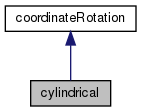[legend]
Collaboration diagram for cylindrical:[legend]

## Public Member Functions

TypeName ("cylindrical")
Runtime type information. More...

cylindrical (const dictionary &, const objectRegistry &)
Construct from dictionary and objectRegistry. More...

cylindrical (const objectRegistry &, const vector &axis, const point &origin)
Construct from components for all cells. More...

cylindrical (const objectRegistry &, const vector &axis, const point &origin, const List< label > &cells)
Construct from components for list of cells. More...

cylindrical (const dictionary &)
Construct from dictionary. More...

cylindrical (const tensorField &)
Construct from tensor Field. More...

autoPtr< cylindricalclone () const
Return clone. More...

virtual ~cylindrical ()
Destructor. More...

virtual void clear ()
Reset rotation to an identity rotation. More...

virtual void updateCells (const polyMesh &mesh, const labelList &cells)
Update the rotation for a list of cells. More...

virtual const tensorR () const
Return local-to-global transformation tensor. More...

virtual const tensorRtr () const
Return global-to-local transformation tensor. More...

virtual const vector e1 () const
Return local Cartesian x-axis in global coordinates. More...

virtual const vector e2 () const
Return local Cartesian y-axis in global coordinates. More...

virtual const vector e3 () const
Return local Cartesian z-axis in global coordinates. More...

virtual const tensorFieldTr () const
Return local-to-global transformation tensor. More...

virtual tmp< vectorFieldtransform (const vectorField &tf) const
Transform vectorField using transformation tensor field. More...

virtual vector transform (const vector &v) const
Transform vector using transformation tensor. More...

virtual vector transform (const vector &v, const label cmptI) const
Transform vector using transformation tensor for component. More...

virtual tmp< vectorFieldinvTransform (const vectorField &vf) const
Inverse transform vectorField using transformation tensor field. More...

virtual vector invTransform (const vector &v) const
Inverse transform vector using transformation tensor. More...

virtual vector invTransform (const vector &v, const label cmptI) const
Inverse transform vector using transformation tensor for component. More...

virtual bool uniform () const
Return if the rotation is uniform. More...

virtual tmp< tensorFieldtransformTensor (const tensorField &tf) const
Transform tensor field using transformation tensorField. More...

virtual tensor transformTensor (const tensor &t) const
Transform tensor using transformation tensorField. More...

virtual tmp< tensorFieldtransformTensor (const tensorField &tf, const labelList &cellMap) const
Transform tensor sub-field using transformation tensorField. More...

virtual tmp< symmTensorFieldtransformVector (const vectorField &vf) const
Transform vectorField using transformation tensorField and return. More...

virtual symmTensor transformVector (const vector &v) const
Transform vector using transformation tensor and return. More...

virtual void write (Ostream &) const
Write. More...Public Member Functions inherited from coordinateRotation
TypeName ("coordinateRotation")
Runtime type information. More...

declareRunTimeSelectionTable (autoPtr, coordinateRotation, objectRegistry,(const dictionary &dict, const objectRegistry &obr),(dict, obr))

declareRunTimeSelectionTable (autoPtr, coordinateRotation, dictionary,(const dictionary &dict),(dict))

virtual ~coordinateRotation ()
Destructor. More...Static Public Member Functions inherited from coordinateRotation
static autoPtr< coordinateRotationNew (const dictionary &dict, const objectRegistry &obr)
Select constructed from dictionary and objectRegistry. More...

static autoPtr< coordinateRotationNew (const dictionary &dict)
Select constructed from dictionary. More...Protected Member Functions inherited from coordinateRotation
symmTensor transformPrincipal (const tensor &, const vector &) const
Transform principal. More...

## Detailed Description

A local coordinate rotation.

The cell based rotational field can be created in two ways:

1. Each rotational tensor is defined with two vectors (`dir` and `e3`) where `dir = cellC - origin` and `e3` is the rotation axis. Per each cell an axesRotation type of rotation is created (cylindrical coordinates). For example:
```      cylindrical
{
type        localAxes;
e3          (0 0 1);
}```
2. The rotational tensor field is provided at construction.
Source files

Definition at line 65 of file cylindrical.H.

## ◆ cylindrical() [1/5]

 cylindrical ( const dictionary & dict, const objectRegistry & obr )

Construct from dictionary and objectRegistry.

Definition at line 96 of file cylindrical.C.

References dictionary::found(), dictionary::lookup(), and dictionary::parent().

Referenced by cylindrical::clone(), and cylindrical::cylindrical().

Here is the call graph for this function: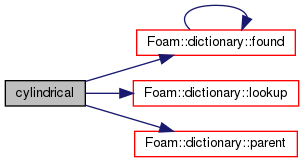Here is the caller graph for this function: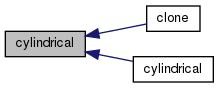## ◆ cylindrical() [2/5]

 cylindrical ( const objectRegistry & obr, const vector & axis, const point & origin )

Construct from components for all cells.

Definition at line 119 of file cylindrical.C.

References cylindrical::cylindrical().

Here is the call graph for this function: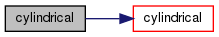## ◆ cylindrical() [3/5]

 cylindrical ( const objectRegistry & obr, const vector & axis, const point & origin, const List< label > & cells )

Construct from components for list of cells.

Definition at line 134 of file cylindrical.C.

## ◆ cylindrical() [4/5]

 cylindrical ( const dictionary & dict )

Construct from dictionary.

Definition at line 149 of file cylindrical.C.

References Foam::exit(), FatalErrorInFunction, and Foam::FatalIOError.

Here is the call graph for this function: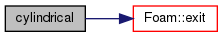## ◆ cylindrical() [5/5]

 cylindrical ( const tensorField & R )

Construct from tensor Field.

Definition at line 165 of file cylindrical.C.

References cylindrical::R().

Here is the call graph for this function: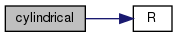## ◆ ~cylindrical()

 virtual ~cylindrical ( )
inlinevirtual

Destructor.

Definition at line 132 of file cylindrical.H.

References cylindrical::clear(), mesh, and cylindrical::updateCells().

Here is the call graph for this function: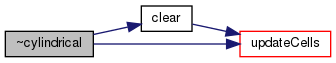## ◆ TypeName()

 TypeName ( "cylindrical" )

Runtime type information.

## ◆ clone()

 autoPtr clone ( ) const
inline

Return clone.

Definition at line 125 of file cylindrical.H.

References cylindrical::cylindrical().

Here is the call graph for this function: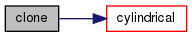## ◆ clear()

 void clear ( )
virtual

Reset rotation to an identity rotation.

Implements coordinateRotation.

Definition at line 177 of file cylindrical.C.

References cylindrical::updateCells().

Referenced by cylindrical::~cylindrical().

Here is the call graph for this function: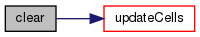Here is the caller graph for this function: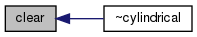## ◆ updateCells()

 void updateCells ( const polyMesh & mesh, const labelList & cells )
virtual

Update the rotation for a list of cells.

Implements coordinateRotation.

Definition at line 187 of file cylindrical.C.

Referenced by cylindrical::clear(), and cylindrical::~cylindrical().

Here is the call graph for this function:Here is the caller graph for this function: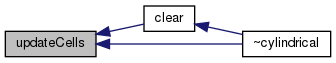## ◆ R()

 virtual const tensor& R ( ) const
inlinevirtual

Return local-to-global transformation tensor.

Implements coordinateRotation.

Definition at line 145 of file cylindrical.H.

Referenced by cylindrical::cylindrical().

Here is the caller graph for this function: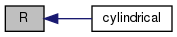## ◆ Rtr()

 virtual const tensor& Rtr ( ) const
inlinevirtual

Return global-to-local transformation tensor.

Implements coordinateRotation.

Definition at line 152 of file cylindrical.H.

Referenced by cylindrical::transformTensor().

Here is the caller graph for this function: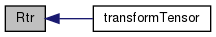## ◆ e1()

 virtual const vector e1 ( ) const
inlinevirtual

Return local Cartesian x-axis in global coordinates.

Implements coordinateRotation.

Definition at line 159 of file cylindrical.H.

References NotImplemented, and VectorSpace< Vector< scalar >, scalar, 3 >::zero.

## ◆ e2()

 virtual const vector e2 ( ) const
inlinevirtual

Return local Cartesian y-axis in global coordinates.

Implements coordinateRotation.

Definition at line 166 of file cylindrical.H.

References NotImplemented, and VectorSpace< Vector< scalar >, scalar, 3 >::zero.

## ◆ e3()

 virtual const vector e3 ( ) const
inlinevirtual

Return local Cartesian z-axis in global coordinates.

Implements coordinateRotation.

Definition at line 173 of file cylindrical.H.

Referenced by cylindrical::write().

Here is the caller graph for this function: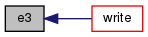## ◆ Tr()

 virtual const tensorField& Tr ( ) const
inlinevirtual

Return local-to-global transformation tensor.

Implements coordinateRotation.

Definition at line 178 of file cylindrical.H.

References cylindrical::invTransform(), tf, and cylindrical::transform().

Here is the call graph for this function: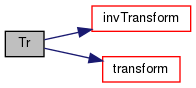## ◆ transform() [1/3]

 Foam::tmp< Foam::vectorField > transform ( const vectorField & tf ) const
virtual

Transform vectorField using transformation tensor field.

Implements coordinateRotation.

Definition at line 207 of file cylindrical.C.

References Foam::abort(), Foam::FatalError, FatalErrorInFunction, and List< T >::size().

Referenced by cylindrical::Tr(), cylindrical::transform(), and cylindrical::updateCells().

Here is the call graph for this function: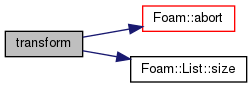Here is the caller graph for this function: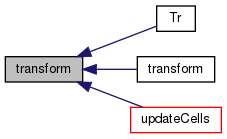## ◆ transform() [2/3]

 Foam::vector transform ( const vector & v ) const
virtual

Transform vector using transformation tensor.

Implements coordinateRotation.

Definition at line 222 of file cylindrical.C.

References NotImplemented, cylindrical::transform(), and Foam::Zero.

Here is the call graph for this function: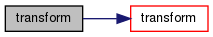## ◆ transform() [3/3]

 Foam::vector transform ( const vector & v, const label cmptI ) const
virtual

Transform vector using transformation tensor for component.

Definition at line 230 of file cylindrical.C.

References cylindrical::invTransform().

Here is the call graph for this function: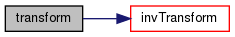## ◆ invTransform() [1/3]

 Foam::tmp< Foam::vectorField > invTransform ( const vectorField & vf ) const
virtual

Inverse transform vectorField using transformation tensor field.

Implements coordinateRotation.

Definition at line 240 of file cylindrical.C.

References Foam::T().

Referenced by cylindrical::invTransform(), cylindrical::Tr(), and cylindrical::transform().

Here is the call graph for this function: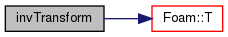Here is the caller graph for this function: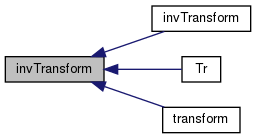## ◆ invTransform() [2/3]

 Foam::vector invTransform ( const vector & v ) const
virtual

Inverse transform vector using transformation tensor.

Implements coordinateRotation.

Definition at line 248 of file cylindrical.C.

References cylindrical::invTransform(), NotImplemented, and Foam::Zero.

Here is the call graph for this function: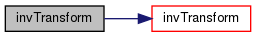## ◆ invTransform() [3/3]

 Foam::vector invTransform ( const vector & v, const label cmptI ) const
virtual

Inverse transform vector using transformation tensor for component.

Definition at line 256 of file cylindrical.C.

References Foam::T(), and cylindrical::transformTensor().

Here is the call graph for this function: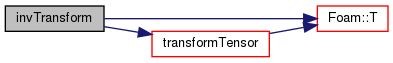## ◆ uniform()

 virtual bool uniform ( ) const
inlinevirtual

Return if the rotation is uniform.

Reimplemented from coordinateRotation.

Definition at line 202 of file cylindrical.H.

Here is the call graph for this function: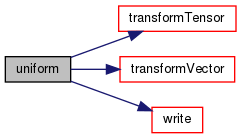## ◆ transformTensor() [1/3]

 Foam::tmp< Foam::tensorField > transformTensor ( const tensorField & tf ) const
virtual

Transform tensor field using transformation tensorField.

Implements coordinateRotation.

Definition at line 266 of file cylindrical.C.

References Foam::abort(), Foam::FatalError, FatalErrorInFunction, List< T >::size(), and Foam::T().

Referenced by cylindrical::invTransform(), cylindrical::transformTensor(), and cylindrical::uniform().

Here is the call graph for this function: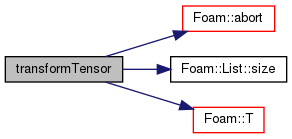Here is the caller graph for this function: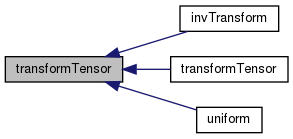## ◆ transformTensor() [2/3]

 Foam::tensor transformTensor ( const tensor & t ) const
virtual

Transform tensor using transformation tensorField.

Implements coordinateRotation.

Definition at line 281 of file cylindrical.C.

References NotImplemented, cylindrical::transformTensor(), and Foam::Zero.

Here is the call graph for this function: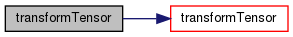## ◆ transformTensor() [3/3]

 Foam::tmp< Foam::tensorField > transformTensor ( const tensorField & tf, const labelList & cellMap ) const
virtual

Transform tensor sub-field using transformation tensorField.

Implements coordinateRotation.

Definition at line 292 of file cylindrical.C.

Here is the call graph for this function: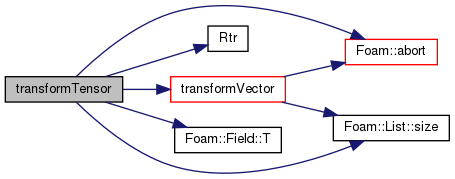## ◆ transformVector() [1/2]

 Foam::tmp< Foam::symmTensorField > transformVector ( const vectorField & vf ) const
virtual

Transform vectorField using transformation tensorField and return.

symmetrical tensorField

Implements coordinateRotation.

Definition at line 319 of file cylindrical.C.

Referenced by cylindrical::transformTensor(), and cylindrical::uniform().

Here is the call graph for this function: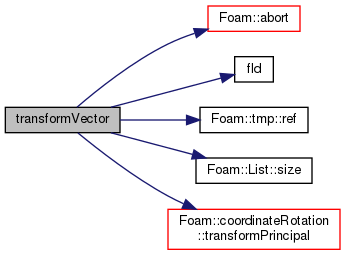Here is the caller graph for this function:## ◆ transformVector() [2/2]

 Foam::symmTensor transformVector ( const vector & v ) const
virtual

Transform vector using transformation tensor and return.

symmetrical tensor (R & st & R.T())

Implements coordinateRotation.

Definition at line 343 of file cylindrical.C.

References NotImplemented, and Foam::Zero.

## ◆ write()

 void write ( Ostream & os ) const
virtual

Write.

Implements coordinateRotation.

Definition at line 352 of file cylindrical.C.

References cylindrical::e3(), token::END_STATEMENT, Foam::nl, and Ostream::writeKeyword().

Referenced by cylindrical::uniform().

Here is the call graph for this function: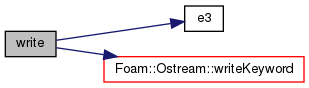Here is the caller graph for this function: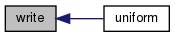The documentation for this class was generated from the following files:
• src/meshTools/coordinateSystems/coordinateRotation/cylindrical.H
• src/meshTools/coordinateSystems/coordinateRotation/cylindrical.C